# ISEE Lower Level Math : Geometry

## Example Questions

### Example Question #1 : Parallelograms

A parallelogram has a side length of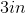.  It also has a side length of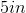.  Calculate the perimeter.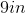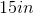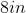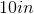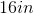Explanation:

A parallelogram has four sides and its opposite sides are equal in length.  Therefore, if it has one side length of, it also has another side length of of.  Since we know one of its side lenghts is, then the remaining side is.  We can add all 4 side lengths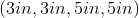to calculate the perimeter.

### Example Question #2 : Parallelograms

What is the perimeter of a parallelogram if the base is, the other side is, and the height is?Explanation:

The perimeter of a parallelogram is the sum of all four sides or the sum of two times each side length.  The side lengths areandso the perimeter is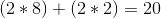.

### Example Question #1 : How To Find The Perimeter Of A Parallelogram

Find the perimeter of the given parallelogram: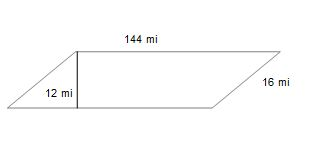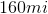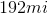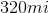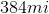Explanation:

Find the perimeter of the given parallelogram:The perimeter of any shape can be found by adding up the lentghs of its sides.

In this case, we have four sides. 2 that are 16 miles long, and 2 that are 144 miles long.

Find perimeter as follows: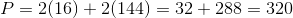Making our answer 320 miles

### Example Question #1 : Plane Geometry

Find the area of the parallelogram: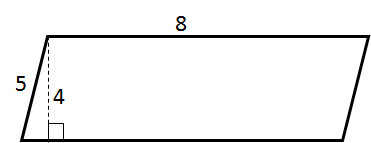Explanation: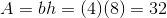### Example Question #1 : Plane Geometry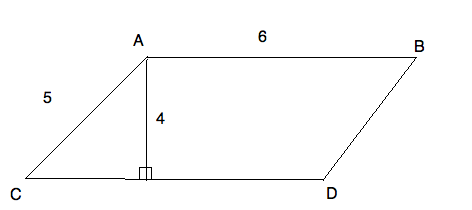What is the area of the parallelogram ABCD?Explanation:

A parallelogram's area is found by multiplying its height by the base. The height of the parallelogram is not the side. It is the line that makes a right angle with the base. Therefore, the area of this parallelogram is: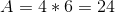### Example Question #3 : Parallelograms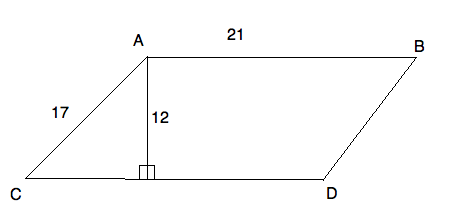What is the area of parallelogram ABCD?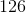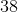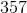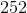Explanation:

A parallelogram's area is found by multiplying its height by the base. The height of the parallelogram is not the side. It is the line that makes a right angle with the base. Therefore, the area of this parallelogram is: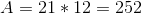### Example Question #4 : Quadrilaterals

A parallelogram measures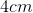along its base and is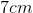high.  Calculate the area.Explanation:

The formula to calucate a parallelogram's area is: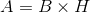You calculate the parallelogram's area by multiplying its base by its height.  Therefore,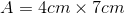### Example Question #1 : Plane Geometry

A parallelogram measures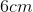along its base and is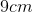high.  Calculate the area.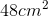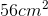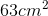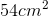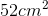Explanation:

The formula to calucate a parallelogram's area is:You calculate the parallelogram's area by multiplying its base by its height.  Therefore,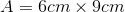### Example Question #6 : Quadrilaterals

Find the area of a parallelogram whose height isand base is.Explanation:

To solve, simply use the formula for the area of a parallelogram. Thus,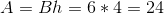### Example Question #11 : Parallelograms

What is the area of a parallelogram if the base is, the other side is, and the height is?The area of a parallelogram is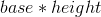so the answer would be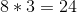.